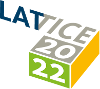#The 39th International Symposium on Lattice Field Theory (Lattice 2022)

Aug 8 – 13, 2022
Hörsaalzentrum Poppelsdorf
Europe/Berlin timezone

## A $(2+1)$-flavor lattice study of the pion quasiparticle in the thermal hadronic phase at physical quark masses

Aug 12, 2022, 3:50 PM
20m
CP1-HSZ/0.011 (CP1-HSZ) - HS1 (CP1-HSZ)

### CP1-HSZ/0.011 (CP1-HSZ) - HS1

#### CP1-HSZ

450
Show room on map
Oral Presentation QCD at Non-zero Temperature

Ardit Krasniqi

### Description

We investigate the properties of the pion quasiparticle in the thermal hadronic phase of $(2+1)$-flavor QCD on the lattice at physical quark masses at a temperature $T = 128\,\text{MeV}$. We find that the pion quasiparticle mass $\omega_{\textbf{0}} = 111(3)\,\text{MeV}$ is significantly reduced relative to the zero-temperature pion mass $m_{\pi}(T=0) = 130(1)\,\text{MeV}$, by contrast with the static screening mass $m_{\pi} = 143(3)\,\text{MeV}$, which increases with temperature. On the other hand the pion quasiparticle decay constant does not change much compared to the corresponding zero-temperature decay constant. The difference of the vector- and axialvector spectral functions serves as an order parameter of chiral symmetry restoration. By analyzing this quantity we conclude that chiral symmetry restoration is already at an advanced stage in the spectral function.

### Primary authors

Ardit Krasniqi Mr Csaba Török (Johannes Gutenberg Universität Mainz) Harvey B. Meyer (Johannes Gutenberg University Mainz) Marco Cè (AEC and ITP, Universität Bern) Tim Harris (University of Edinburgh)